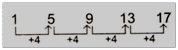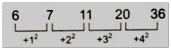# IBPS Specialist Officers Exam: Reasoning-Series-Concept & Sample Questions

Institute of Banking and Personnel Selection (IBPS) will organize common written examination for recruitment of Specialist Officers. The IBPS SO Exam 2015 will be conducted in the month of February 2015.

Created On: Dec 30, 2014 16:45 IST
Modified On: Jan 19, 2015 12:35 ISTInstitute of Banking and Personnel Selection (IBPS) will organize common written examination for recruitment of Specialist Officers. The IBPS SO Exam 2015 will be conducted in the month of February 2015.The banking team of jagranjosh.com has come up with concept and sample questions for Series (Reasoning). The concept provided by us will help you to understand the topic. The sample questions offered by us are framed by keeping in view need of the question paper.

Type 1 Inserting a number

Here, we are give a sequence of numbers this sequence has one missing number. We have to understand the pattern and then find the missing number, some possible cases can be understand by given examples.

Examplel 1. 1    5    9     13 ?

(a) 14

(b) 15

(c) 16

(d) 18

(e) None of these

Solution: - (e) Clearly the give sequence follows the pattern + 4, + 4, + 4,....., 0

Now, missing term = 13 + 4 = 17Example 2. 6    7    11    20 ?

(a) 25

(b) 132

(c) 36

(d) 48•

(e) Noneofthese

Solution: - (c) Clearly the given sequence follows the pattern, + 12, + 22, + 32, + 42.

Now, missing term = 20 + 42 = 20 + 16 = 36Type 2 wrong number Series

Such sequence consists a collection of numbers; one out of them is placed wrong. Following the pattern, we have to detect the wrong term.

Example 3. 1   3    12   25    48

(a) 3

(b) 12

(c) 25

(d) 48

(e) None of these

Solution: - (c) The terms of the series are (12 - 02); (22 - 12); (42 - 22); (62 - 32) and (82 - 42).

So, 25 is wrong and must be placed by (62 - 32) = 36 - 9 = 27

Example 4. 16   19    21    30    46    71    107

(a) 19

(b) 21

(c) 30

(d) 46

(e) 71

Solution: - (a) The series is based on the following pattern

1O7 – 71 = 36 = 62, 71 – 46 = 25 = 52, 46 - 30 = 16 = 42

30 – 21 = 9 = 32, 21 – 19 = 2 ≠ 22

So, 19 should be replaced by 17 for which 21 - 17 = 22

Type 3 Having Two Series

Here, we are given two series. One series is complete and another is incomplete. We have to study first complete series and then have to detect the pattern, then this pattern is to be applied with incomplete series.

Directions (Examples 5): - In each of the following questions a number series is given. After the series a number is given followed by (a), (b), (c), (d) and (e). You have to complete the series starting with the number given, following the sequence of the original series and answer the questions that foliow the series.

5. 12    30    120    460    1368   2730
16    (a)     (b)       (c)       (d)       (e)

What will come in place of (d)?

(a) 1384

(b) 2642

(c) 2808

(d) 1988

(e) None of these

Solution: - (c) The given series is based on the following pattern

30 = 12 x 6 – 7 x 6,

120 = 30 x 5 – 6 x 5

460 = 120 x 4 – 5 x 4

1368 = 460 x 3 – 4 x 3

2730 = 1368 x 2 – 3 x 2

Similarly,

(a) = 16 x 6 – 7 x 6 = 96 – 42 = 54

(b) = 54 x 5 – 6 x 5 = 270 – 30 = 240

(c) = 240 x 4 – 5 x 4 = 960 – 20 = 940

(d) = 940 x 3 – 4 x 3 = 2820 – 12 = 2808

Hence, 2808 will come in place of (d).

Sample Questions with Solutions and Explanations